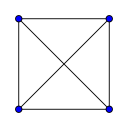# Queries, Queries Everywheresalman.exeCoU-BRACNet Inter Univers...53/58/163
Limits 1s, 512 MB

You are given an array $a$ of length $n$. You are also given $q$ queries on the array and you have to process them. Queries are of two types:

• $1~~i~~v$: Set $a[i]=v$.

• $2~~L~~R$: Construct a complete graph on exactly $R-L+1$ nodes $L,L+1,\ldots,R$. The graph is complete, meaning every node is connected to every other node by an edge. The weight of the edge between two nodes is the maximum of their values. Formally, the edge between nodes $u,v$ is $\max(a[u],a[v])$, where $L\leq u. You have to print the cost of the minimum spanning tree (MST) of this graph.

Here is an example of an unweighted complete graph with $4$ nodes (note that in our case the edges will have weights).A spanning tree of a graph is a subset of edges taken from the graph such that they form a tree and every vertex on the graph is reachable from every other vertex via the chosen set of edges. The cost of a spanning tree is just the sum of edge weights in it. A minimum spanning tree of a graph is a spanning tree with the minimum cost.

## Input

The first line contains the integer $T$ $(1 \leq T \leq 100000)$, the number of test cases. For each test case:

The first line contains the integers $n$ $(1 \leq n \leq 200000)$ and $q$ $(1 \leq q \leq 100000)$.

The second line contains $n$ integers describing the contents of the array $a$ $(1 \leq a[i] \leq 2\times 10^9)$.

The next $q$ lines are the queries you have to process in one of the following forms:

• $1~~i~~v$: These integers satisfy $1 \leq i \leq n$ and $1 \leq v \leq 2\times 10^9$. In this case, you have to set $a[i]=v$.

• $2~~L~~R$: These integers satisfy $1\leq L\leq R\leq N$. In this case, you have to print the cost of the MST in the graph described above.

The sum of $n$ over all test cases does not exceed $2\times 10^5$. The sum of $q$ over all test cases does not exceed $10^5$.

## Output

For each query of the second type, output a single number in one line - the cost of the MST of the graph constructed.

Don’t print an extra newline after each test case. Every case has at least one query of the second type.

## Sample

InputOutput
1
5 6
1 2 3 4 5
1 1 9
1 2 8
1 3 3
1 4 3
1 5 11
2 2 5

22


There can be multiple ways to construct an MST.

### Submit